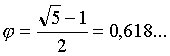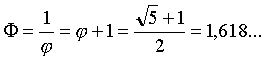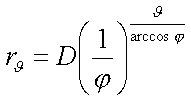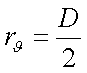# Chap.3.1 The Cosmological Equation.

## Chapter 3.1  The Cosmological Equation.

§ 1. RELATIONSHIP BETWEEN TWO GRAVITATIONAL SOURCES.

In chap.1.9 we have been talking about a fundamental relationship in between the law of gravitation, and hence in our “cosmological equations”. We have been referring to it as the relatonship between peripheral mass and central mass. Actually the meaning of the term mass in contemporaruy physics is radically different from the one in unigravitational physics. For us it is only the first factor of the universal formula: this replaces it with a value that includes other factors in the broader term gravitational strength. It is precisely these other factors, ignored by the mainstream physics as universal attributes of gravitation, which makes the value of the intensity of gravity in relation to the bare mass grow exponentially in the microcosm – where they are prominent – suggesting the existence of forces other than gravity in the subatomic world. The mass has, in fact, in our physics, the the more logical and older meaning of amount of substance, ie more simply the number of particles constituting a body. Yet we know that contemporary physics has transformed the mass, with relativity, into an abstract mathematical concept variable with the speed of the body: which has no physical sense, as shown by a correct reading of what is cited as evidence of this variability (chap.1.3).

It ‘goes without saying that the intensity of gravity should not be confused with the gravitational force, which is the effect of mutual attraction between two bodies interacting, while the’ “intensity” is a value inherent in each body.

We can express with a’/ a the above relation, being a’ and a the gravitational intensity of two interacting material structures – not necessarily two bodies identified as separate – or two gravitational sources, so that

0 <= a’ <= a                                      with  a > 0                                     and then                                            0 <= a’ / a <= 1 .

In other words, a is the gravitational source of reference, always > 0 for intensity; a’ has an intensity value ranging from 0 (only theoretical absence of a second source) to the value in itself (“equintensity” of the two sources).

The geometry of the structures of the universe does not depend on the absolute values ​​of the intensity of the two interacting sources but only from the report a’/ a, which – as noted above – varies from 0 to 1.

§ 2. GOLDEN SECTION AND GOLDEN RATIO.We attach the symbol φ to the value of the “golden section”, so that the actual geometry and mathematics characteristcs refer to the relevant disciplines.The symbol φ  represents the “golden ratio”, which is the inverse of the golden section and also the same increased by 1.

The Golden Section, or its inverse in nature, are the basis of the “Fibonacci sequence” (1,1,2,3,5,8,13,21, …: each number is the sum of the previous two and the ratio of two successive numbers tends to infinity to the golden value) of penta-decagonal and dodeca-icosahedron figures, the factor of increase of spirals forms for equal angles of rotation: this in phyllotaxis, in crystallography, molecular biology, etc.. . There is a vast literature on this popular subject, but no naturalistic explanation beyond mere description of this morphology.

§ 3. “CONJUGATION” BETWEEN TWO GRAVITATIONAL SOURCES.

In sec. 1 we discussed the waving conjugation as an exclusive faculty of a spherical eccentric propagation, rotating around a non- central center of gravity. Two or more eccentric propagations can bring together their pairs of spherical waves in a single geometric spherical wave of coincidence of two or more waves departing from different gravitational sources. The wave of coincidence (composed wave) sums the intensities of the parent gravitational sources, but soon becomes a primitive third propagation wave (“daughter propagation”) and divides the sum of the accumulated intensities between parent and child, according to a mathematical law we will talk about later.

Each gravitational field, but the basic one (photonic gravitational field), arises from the conjugation between different propagation waves, with a primitive spherical propagation wave and a basic diameter among the infinite potentials fixed by a precise law of composition that we will give later. The extremes of this diameter are the two sources of gravitational field, covered by the cosmological equation, and of course the diameter is the distance himself. For this equation we give the symbol D.

This means that the problem that haunts the mainstream physics concerning the interaction of two more bodies is theoretically solved by combining i couples two sources from less intense to more intense, and the resulting wave with another one, up to the source of greatest intensity.

§ 4. THE CENTER OF GRAVITY OF PROPAGATION.

The fraction  Da / (a+a’) divides the diameter D of the wave into two parts inversely proportional to a’ and a, measuring the diameter having as an extreme a’.

So if a’ is zero (only in the basic field, or – roughly – when the source a is vastly more intense than a’), the fraction equals D and the center of gravity coincides with the more intense source, which is also the only one ( the photon, or – roughly – the source of greatest intensity from a source of considerable intensity, distant a D lengh from the predominant one).

If a’= a, ie if the two sources are equintense, the fraction equals D/2: ie, the centre of gravity divides the diameter into two equal parts, which are rays of the resulting wave.

§ 5. THE PROPAGATION SPIRAL.

We can call A and A’ the extremes of the diameter D of the basic wave, in their correspondence of a and a’. The wave expands spherically, but also rotating around the center of gravity of propagation (Fig.1  in chap.1.9). If this is not central, so if a and a’ are not equintense (unequintense), expansion and rotation of the wave are regulated by the cosmological wave so that A’ and A follows two logarithmic spirals with constant linear velocity: which implies that A’ is faster than A, but each of the two extremes will cover equal ground spiral arcs in equal times. For the properties of the logarithmic spiral, the linear increment of spiral rays are also equal and within equal times (the diameter D is divided by the center of gravity into a pair of spiral-rays: the bigger with A’ as an extreme, and the smaller with extreme A).

The rotation of the wave determines a perpendicular axis of rotation among the center of gravity to a plane of rotation, which cuts the rotating wave into two hemispheres, separated by an equatorial circle lying on the plane of rotation. The poles of the axis of rotation is conventionally the North, the one that sees the counterclockwise rotation of the wave, and the South, who sees the clockwise one.

§ 6. THE COSMOLOGICAL EQUATION.

It’s the mathematics of unigravitational physics, inseparable from it, as well as the mathematical basis of the OLOPOIEMA software.

The cosmological equation gives the value of the greater spiral ray, for any Θ  (theta) rotation, and then builds the propagation of gravity in relation to D, a and a’.

We will demonstrate that the matter comes together and is structured, both in macro-and microcosm, according to this one equation. We begin here with to be able to appreciate  the two equation’s limit natural effects. This will mean, in itself, a confirmation of the universality of it.

1)     For a’= 0, ie when the bigger gravitational source tends to prevail over the smaller one (in theory without an end, and in elementary field – the photonic one – also in absolute terms, for the lack of an a’ source), the ‘equation above equalsSo that rθ grows according to the exponentiation Θ /arccosφ of 1/φ , or of Φ (§ 2).

This first limit, represented by the minimum value of a’, gave the mathematical reason of all the structures related to the golden section, which we mentioned in § 2 and whose mainstream science ignores any motivation, only knowing that they are very frequent in nature.

The angle arccosφ, ie the angle whose cosine is φ , is 51.827292 … degrees.

2)     For a’= a (maximum value of a’), ie when the gravity sources are equintense, the cosmological equation is insteadThis means that, for any rotation, the radius rΘis always equal to the half of D, and is therefore the radius of a circle. Clearly this is the mathematical reason of all natural forms of circular or spherical type.

At this point it is even obvious – but we shall see this in details in the next page – that, for the infinite intermediate values of a’/ a, our equation will draw all the other aggregative structures existing in nature, biological or not, hiding the value of the golden section within the intermediate cases, which appears so haphazard, but which represents the foundation of the universe.